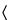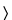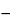Aluminum Zirconium Tetrachlorohydrate Solution
» Aluminum Zirconium Tetrachlorohydrate Solution is a polymeric, loosely hydrated complex of basic aluminum zirconium chloride that encompasses a range of aluminum-to-zirconium atomic ratios between 2.0:1 and 5.99:1 and a range of (aluminum plus zirconium)-to-chloride atomic ratios between 1.5:1 and 0.9:1. The following solvents may be used: water, propylene glycol, or dipropylene glycol. It contains the equivalent of not less than 90.0 percent and not more than 110.0 percent of the labeled concentration of anhydrous aluminum zirconium tetrachlorohydrate.
Packaging and storage— Preserve in well-closed containers.
Labeling— Label Solution to state the solvent used and the claimed concentration of anhydrous aluminum zirconium tetrachlorohydrate.
Identification—
A: A solution containing the equivalent of about 100 mg of anhydrous aluminum zirconium tetrachlorohydrate per mL responds to the test for Chloride191.
B: Identification of propylene glycol (where stated on the label)—Add about 10 mL of isopropyl alcohol to 2 g of Solution, mix, and filter. Evaporate the filtrate to about 1 mL on a steam bath: the IR spectrum of a film of this solution on a silver chloride disk exhibits maxima only at the same wavelengths as that of a similar preparation of a film of propylene glycol.
C: Identification of dipropylene glycol (where stated on the label)—Add about 10 mL of isopropyl alcohol to 2 g of Solution, mix, and filter. Evaporate the filtrate to about 1 mL on a steam bath: the IR spectrum of a film of this solution on a silver chloride disk exhibits maxima only at the same wavelengths as that of a similar preparation of a film of dipropylene glycol.
between 3.0 and 5.0, in a solution prepared by diluting 3 g of the Solution with water to obtain 10 mL.
Prepare the Test Preparation using an accurately weighed quantity of the Solution. The limit is 2 µg per g.
Proceed as directed in the chapter, except to use the following modifications.
Test Preparation— Prepare as directed in the chapter, using an accurately weighed quantity of Solution. If the solution is not clear after dilution to 40 mL, heat for several minutes between 60and 80, and cool to room temperature. If the solution remains cloudy, repeat from the beginning with the following modification: add 3 mL of hydrochloric acid prior to adjustment of the pH with 6 N ammonium hydroxide.
Monitor Preparation— Prepare as directed in the chapter, using the same modifications described for Test Preparation if necessary.
Procedure— To each of the three tubes containing the Standard Preparation, the Test Preparation, and the Monitor Preparation, add 2 mL of pH 3.5 Acetate Buffer, and heat gently to between 60and 80. To the Standard Preparation add 6 drops of sodium sulfide TS. To the Test Preparation and the Monitor Preparation add 12 drops of sodium sulfide TS. Cool to room temperature, and dilute the contents of each tube with water to 50 mL. Gently mix each tube by inverting twice. Allow to stand for 5 minutes, and view downward over a white surface. The color of the solution from the Test Preparation is not darker than that of the solution from the Standard Preparation, and the color of the solution from the Monitor Preparation is the same as or darker than the color of the solution from the Standard Preparation. If the color of the solution from the Monitor Preparation is lighter than the color of the solution from the Standard Preparation, repeat the procedure with the following modification: after the heating step, to the Monitor Preparation and the Test Preparation add 1.0 mL, instead of 12 drops, of sodium sulfide TS. Not more than 10 µg per g is found.
Limit of iron— Using about 5.4 g of Aluminum Zirconium Tetrachlorohydrate Solution, accurately weighed, instead of Aluminum Zirconium Octachlorohydrate, proceed as directed in the test for the Limit of iron under Aluminum Zirconium Octachlorohydrate. The limit is 75 µg per g.
Content of aluminum— Using about 0.3 g of Aluminum Zirconium Tetrachlorohydrate Solution instead of Aluminum Zirconium Octachlorohydrate, proceed as directed in the test for the Content of aluminum under Aluminum Zirconium Octachlorohydrate. Use the result to calculate the Aluminum/zirconium atomic ratio and the (Aluminum plus zirconium)/chloride atomic ratio.
Content of zirconium— Using about 500 mg of Aluminum Zirconium Tetrachlorohydrate Solution, accurately weighed, instead of Aluminum Zirconium Octachlorohydrate, proceed as directed in the test for the Content of zirconium under Aluminum Zirconium Octachlorohydrate. Use the result to calculate the Aluminum/zirconium atomic ratio and the (Aluminum plus zirconium)/chloride atomic ratio.
Aluminum/zirconium atomic ratio— Divide the percentage of aluminum found in the test for Content of aluminum by the percentage of zirconium found in the test for Content of zirconium and multiply by 92.97/26.98, in which 92.97 is the atomic weight of zirconium corrected for 2% hafnium content, and 26.98 is the atomic weight of aluminum: the ratio is between 2.0:1 and 5.99:1.
Content of chloride— Using about 500 mg of Aluminum Zirconium Tetrachlorohydrate Solution, accurately weighed, instead of Aluminum Zirconium Octachlorohydrate, proceed as directed in the test for the Content of chloride under Aluminum Zirconium Octachlorohydrate. Use the result to calculate the (Aluminum plus zirconium)/chloride atomic ratio.
(Aluminum plus zirconium)/chloride atomic ratio— Calculate the (aluminum plus zirconium)/chloride atomic ratio by the formula:
[(Al/26.98) + (Zr/92.97)]/(Cl/35.453)
in which Al, Zr, and Cl are the percentages of aluminum, zirconium, and chloride found in the test for Content of aluminum, Content of zirconium, and Content of chloride, respectively; 26.98 is the atomic weight of aluminum; 92.97 is the atomic weight of zirconium corrected for 2% hafnium content; and 35.453 is the atomic weight of chlorine: the ratio is between 1.5:1 and 0.9:1.
Assay— Calculate the percentage of anhydrous aluminum zirconium tetrachlorohydrate in the Solution by the formula:
Al({26.98y + 92.97 + 17.01[3y + 4(y + 1)/z] + 35.453(y + 1)/z}/26.98y)
in which Al is the percentage of aluminum found in the test for Content of aluminum, y is the aluminum/zirconium atomic ratio found in the test for Aluminum/zirconium atomic ratio, z is the (aluminum plus zirconium)/chloride atomic ratio found in the test for (Aluminum plus zirconium)/chloride atomic ratio, 26.98 is the atomic weight of aluminum, 92.97 is the atomic weight of zirconium corrected for 2% hafnium content, 17.01 is the molecular weight of the hydroxide anion (OH), and 35.453 is the atomic weight of chlorine (Cl).
Auxiliary Information— Please check for your question in the FAQs before contacting USP.
Topic/Question Contact Expert Committee
Monograph Feiwen Mao, M.S.
Senior Scientific Liaison
1-301-816-8320
(SM32010) Monographs - Small Molecules 3
USP35–NF30 Page 2146
Pharmacopeial Forum: Volume No. 27(4) Page 2697